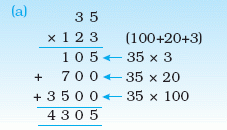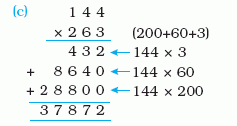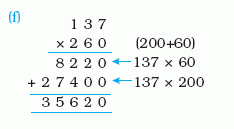Email us to get an instant 20% discount on highly effective K-12 Math & English kwizNET Programs!

#### Online Quiz (WorksheetABCD)

Questions Per Quiz = 2 4 6 8 10

### Grade 4 - Mathematics3.27 Multiplication - Three Digits (WIZ Math)

 Multiplying 2 digit and 3 digit numbersMultiplying 3 digit and 2 digit numbersMultiplying 3 digit and 3 digit numbersDirections: Multiply the numbers shown in the question. Use a separate sheet to show your work and write the answer in the box.
 Question 1: 788 * 892

 Question 2: 491 * 851

 Question 3: 521 * 98

 Question 4: 481 * 438

 Question 5: 506 * 17

Question 6: This question is available to subscribers only!

Question 7: This question is available to subscribers only!

Question 8: This question is available to subscribers only!

Question 9: This question is available to subscribers only!

Question 10: This question is available to subscribers only!